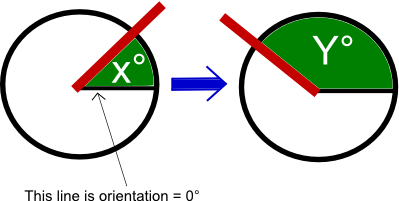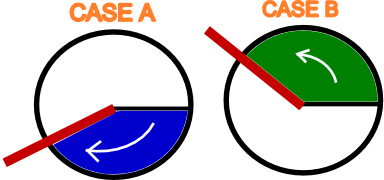When you build some animations with WPF, Surface or JavaFX you sometimes need to know how evolve an angle. For example, you have the new angle (orientation) of an object and you have store before the last measure of this orientation : how to calculate the evolution ?## A first solution

"This is simple" will you say, just do this :

`double difference = secondAngle - firstAngle;`

But this snippet leads to errors. This is because the orientation value given is relative to a certain initial value, and restart to 0° if you pass the 360°. Lets give you an example: if the object was oriented at 358° and the user turns it of 5° you will obtain a difference of (3-358=) -355° where you actually wants to find 5....

## A better solution

A solution I propose is to consider that the methods is called enough frequently that if the object rotate to the left or to the right it has not enough time to rotate more than 180° (half a tour).

Based on this, we consider that the direction of the rotation is given by the "shortest way". If it shorter to turn to the left to go to the new angle, then we select the angle sign which tells we have turned to the left. It may be easiest to understand by looking atentivly to the image above.

An image is maybe better than word :We then have this method in C#:

```private double calculateDifferenceBetweenAngles(double firstAngle, double secondAngle)
{
double difference = secondAngle - firstAngle;
while (difference < -180) difference += 360;
while (difference > 180) difference -= 360;
return difference;
}```

## A case of use

When do use it ? For example when you build a carousel: the user can click on a specific item and the carousel rotate so it is in front of you. You then need to have the correct angle. I found no other way to do it.

### Does someone have a better way to proceed ?